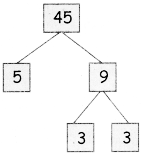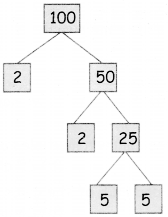Students can download 5th Maths Term 2 Chapter 2 Numbers Additional Questions and Answers, Notes, Samacheer Kalvi 5th Maths Guide Pdf helps you to revise the complete Tamilnadu State Board New Syllabus, helps students complete homework assignments and to score high marks in board exams.

## Tamilnadu Samacheer Kalvi 5th Maths Solutions Term 2 Chapter 2 Numbers Additional Questions

Question 1.
Find the common factors for:
(i) 6 and 9
(ii) 15 and 20
(i) 6 and 9
Factors of 6 is 1, 2, 3, 6
Factors of 9 is 1, 3, 9
Common factors of 6 and 9 is 1, 3

(ii) 15 and 20
Factors of 15 is 1, 3, 5, 15
Factors of 20 is 1, 2, 4, 5, 10, 20
Common factors of 15 and 20 are 1, 5.Question 2.
Draw a picture of factor tree.
(i) 45
(ii) 100
(i) 45(ii) 100Question 3.
Write down the first 5 multiple of the given numbers.
(i) 15
(ii) 25
(i) 15
15 × 1 = 15
15 × 2 = 30
15 × 3 = 45
15 × 4 = 60
5 × 5 = 75
5, 30, 45, 60, 75(ii) 25
25 × 1 = 25
25 × 2 = 50
25 × 3 = 75
25 × 4 = 100
25 × 5 = 125
25, 50, 75, 100, 125